# Algorithmic Differentiation Background¶

As every computer program is made up of a series of simple arithmetic operations, i.e.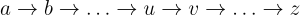where the inputs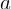are modified in stages in order to get the final output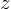. When the individual derivatives of each operation are known, the final derivative can be computed by recursive application of the chain rule. This is method is called Algorithmic Differentiation, with the modes forward (or tangent-linear), i.e., from inputs to outputs, and adjoint (or reverse) mode, i.e., from outputs to inputs.

In this section, we introduce the underlying theory for computing derivatives of a computer program. We start with a review of the traditional finite difference method, often called bumping, before introducing forward and adjoint algorithmic differentiation.

## Finite Differences¶

The traditional approach for computing these derivatives is by employing a finite difference approximation. That is, each of the input variables are bumped one by one and the change of the result is used to estimate the sensitivities:where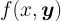is the function of which we are interested in derivatives with respect to the input parameter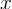. The vector-valued argument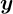denotes the remaining function parameters. The first equation represents forward finite differences and requires two evaluations of the function. The second equation gives central finite differences with potentially higher accuracy and requires two function evaluation for the derivative and another evaluation for the function’s value.

In practice, the value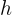is chosen small enough to approximate the theoretical limit, but large enough to cause a detectable change of the result beyond typical numerical error levels. Clearly, this choice impacts the accuracy of the approximation.

Further, this method implies that the function needs to be evaluated once for the result and once for each derivative that we are interested in. This results in a high overall computational complexity as soon as more than a few derivatives are needed.

Thus, the finite differences approach has accuracy and performance limitations.

## Forward Mode¶

### Theory¶

The forward mode defines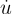as the derivative of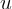with respect to, i.e.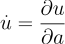Applying the chain rule of differentiation and assuming that the intermediate variables are vectors, the elements of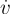can be calculated as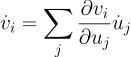Applying this to each step in the chain of operations from inputs to outputs, the value of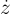can be calculated. This is the forward mode of algorithmic differentiation.

For a function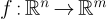, one application of the forward mode gives the sensitivities for all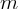outputs with respect to one input parameter. It needs to be re-evaluated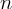times to obtain all sensitivities. The computational cost is constant in the number of output variablesand linear in the number of input variables.

### Example¶

We illustrate the forward mode on the example function: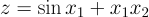Which can be implemented in a computer program as:

a = sin(x1);
b = x1 * x2;
z = a + b;


We are interested of the derivative with respect to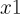for the input values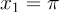and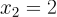. The following figure illustrates how the forward mode algorithm differentiation is applied to this problem:On the left we see the computational graph representing the equation, and the table on the right illustrates the the steps performed.

In step 0, we initialize the input values and we seed the derivatives of these inputs. As we are interested in the derivative w.r.t.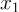, we set its derivative to 1 while setting the other to 0.

Next we computeby taking the sine function. The value ofis zero, while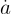is computed by multiplying the partial derivative of the sine w.r.t. to, i.e. the cosine, with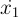. This gives a value of -1.

In the next step, the value of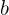is computed as usual, and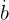is calculated similarly to, this time depending on bothand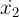. This results in a value of 2.

The final statement adds bothand, which gives the result of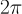. To calculate, we see that theandcan simply be added since their partial derivatives are both 1. This gives a final derivative of 1.

Hence: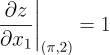which can be easily verified analytically.

### Theory¶

The adjoint mode applies the chain rule backwards, from outputs to inputs. Using standard notation, we define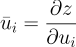whereis the index in the vector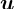. Applying the chain rule yields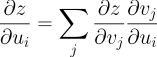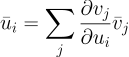Seeding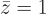, the adjoint mode equation can be applied for each step, from output to input, to obtain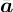, which is the derivative of the outputwith respect to each of the input variables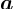.

For a function, the adjoint mode gives the sensitivities of one output with respect to allinput parameters. It needs to be re-evaluatedtimes to obtain all sensitivities. The computational cost is constant in the number of input variablesand linear in the number of output variables.

### Example¶

We illustrate the adjoint mode using the same example as above:implemented:

a = sin(x1);
b = x1 * x2;
z = a + b;


With adjoint mode, we can get both partial derivatives of the output in a single execution, for the input valuesand. This is illustrated in the figure below:As the adjoint mode walks from outputs back to inputs, we execute the full computation of the value as usual, until we have an output forof.

Then we seed the adjoint ofto 1 in the final step, and walk backwards to compute the adjoints of the inputs.

In step 2, we can compute the adjoint ofby multiplying the adjoint ofwith the partial derivative of the equation forw.r.t., which is 1.

The same is performed in step 1 to compute the adjoint of, which also yields 1.

The adjoint of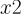is then computed by multiplying the partial derivative ofw.r.t.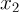with the adjoint of, which gives the value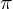.

The same method is applied to compute the adjoint of, giving the value 1.

Thus, the two derivatives we were interested in are:Which can be easily verified analytically.

## Higher Orders¶

Higher order derivatives can be obtained by nesting the principles described above. For example, applying forward mode algorithmic differentiation over adjoint mode gives second order derivatives. This method can be extended to any order.

Last update: February 2023